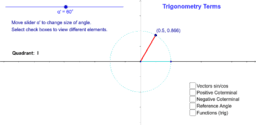•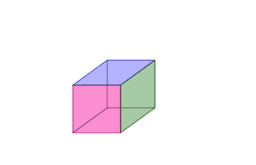x^3 small

Activity

•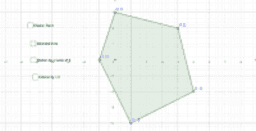Dilation on a graph

Activity

•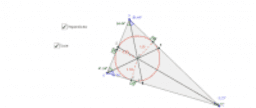Angle bisector triangle 1

Activity

•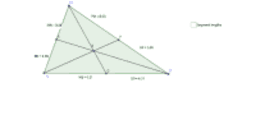Medians Triangle

Activity

•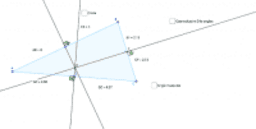Perpendicular bisector triangle

Activity

•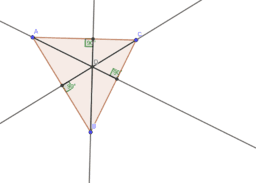Altitudes

Activity

•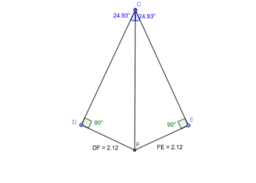Angle Bisector Theorem

Activity

•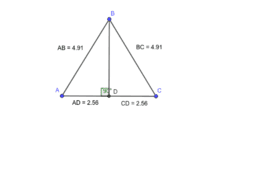Converse of the Perpendicular Bisector Theorem

Activity

•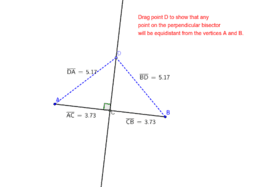Copy of Perpendicular bisector/endpoints theorem #2

Activity

•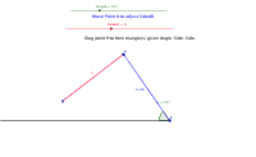Copy of SSA Testing Triangle Congruence ? Angle-Side-Side ?

Activity

•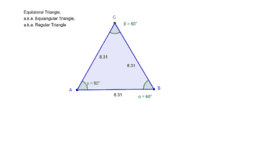Copy of Equilateral Triangle

Activity

•Copy of Isosceles Triangle

Activity

•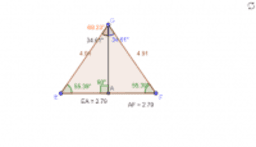Line bisects vertex angle of isosceles triangle

Activity

•Side Angle Side

Activity

•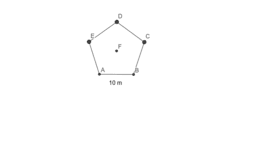Finding area

Activity

•